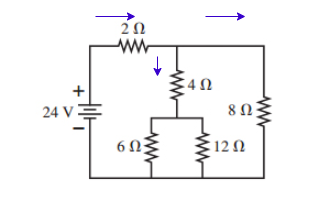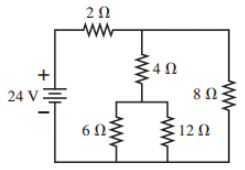# Problem: For the circuit shown in the figure:(a) Find the current through each resistor.(b) Find the potential difference across each resistor.

###### FREE Expert Solution
97% (314 ratings)
###### FREE Expert SolutionResistors in series:

$\overline{){{\mathbf{R}}}_{\mathbf{e}\mathbf{q}}{\mathbf{=}}{{\mathbf{R}}}_{{\mathbf{1}}}{\mathbf{+}}{{\mathbf{R}}}_{{\mathbf{2}}}{\mathbf{+}}{\mathbf{.}}{\mathbf{.}}{\mathbf{.}}{\mathbf{+}}{{\mathbf{R}}}_{{\mathbf{n}}}}$

Resistors in parallel:

$\overline{)\frac{\mathbf{1}}{{\mathbf{R}}_{\mathbf{e}\mathbf{q}}}{\mathbf{=}}\frac{\mathbf{1}}{{\mathbf{R}}_{\mathbf{1}}}{\mathbf{+}}\frac{\mathbf{1}}{{\mathbf{R}}_{\mathbf{2}}}{\mathbf{+}}{\mathbf{.}}{\mathbf{.}}{\mathbf{.}}{\mathbf{+}}\frac{\mathbf{1}}{{\mathbf{R}}_{\mathbf{n}}}}$

Current:

$\overline{){\mathbf{i}}{\mathbf{=}}\frac{\mathbf{V}}{\mathbf{R}}}$

97% (314 ratings)###### Problem Details

For the circuit shown in the figure:
(a) Find the current through each resistor.
(b) Find the potential difference across each resistor.Frequently Asked Questions

What scientific concept do you need to know in order to solve this problem?

Our tutors have indicated that to solve this problem you will need to apply the Solving Resistor Circuits concept. You can view video lessons to learn Solving Resistor Circuits. Or if you need more Solving Resistor Circuits practice, you can also practice Solving Resistor Circuits practice problems.

What professor is this problem relevant for?

Based on our data, we think this problem is relevant for Professor Markert's class at TEXAS.